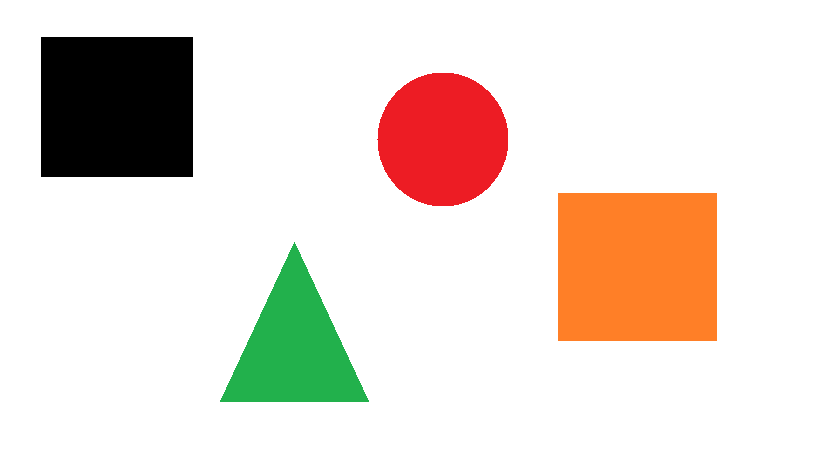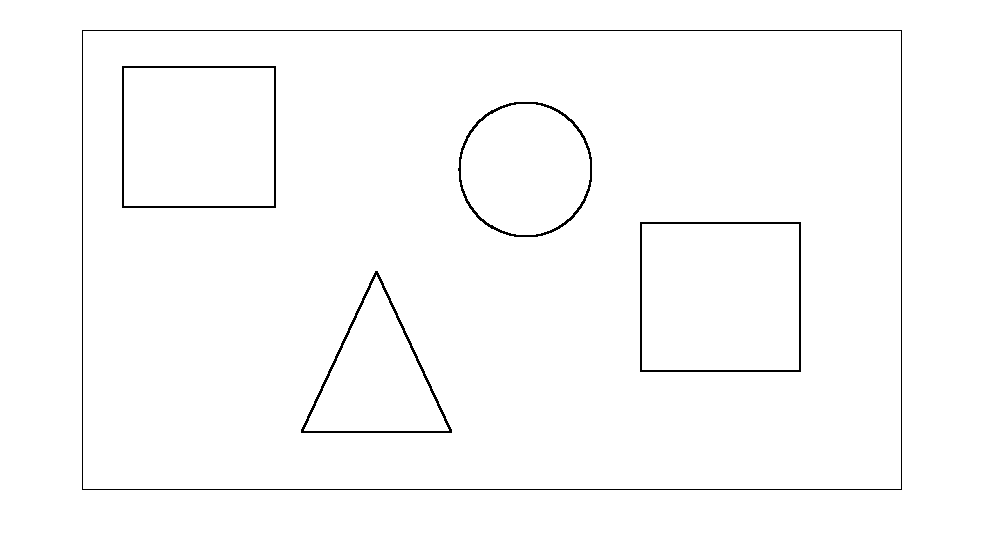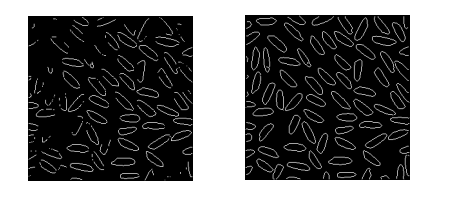# Edge Detection

#### Introduction

As the name suggests, edge detection is a means to locate the edges of objects in a particular image. This is a fundamental image processing technique for detecting corners, sharp turns, and in identifying shapes. Edge detection has a lot of scope for precision and efficiency, and there are many well-developed algorithms to deal with it. Here we will be studying a basic conversion which will help us understand the concepts involved.

In this program, we use the gray-scale equivalents of the pixels, which represent the intensity of the pixel, for our edge detection. We cycle through each pixel, and check all the 8 pixels around it, in a sub-unit of 3X3. We store the maximum and minimum pixel intensities. If the difference between the highest and the lowest value is greater than a particular threshold, then that particular pixel is considered to belong to an edge, and in the resultant image, we designate as black (part of an edge). Otherwise we designate it white. The final edge-detected image is a binary image where the black portions represent the edges.#### Using Functions in the IPT

You can use the edge function to detect edges, which are those places in an
image that correspond to object boundaries. To find edges, this function looks
for places in the image where the intensity changes rapidly, using one of these
two criteria:

•Places where the first derivative of the intensity is larger in magnitude than
some threshold
•Places where the second derivative of the intensity has a zero crossing

edge provides a number of derivative estimators, each of which implements one
of the definitions above. For some of these estimators, you can specify whether
the operation should be sensitive to horizontal or vertical edges, or both. edge
returns a binary image containing 1’s where edges are found and 0’s elsewhere.
The most powerful edge-detection method that edge provides is the Canny
method. The Canny method differs from the other edge-detection methods in
that it uses two different thresholds (to detect strong and weak edges), and
includes the weak edges in the output only if they are connected to strong
edges. This method is therefore less likely than the others to be “fooled” by
noise, and more likely to detect true weak edges.

The example below illustrates the power of the Canny edge detector. It shows
the results of applying the Sobel and Canny edge detectors to the rice.tif
image.B1                                                                     B2